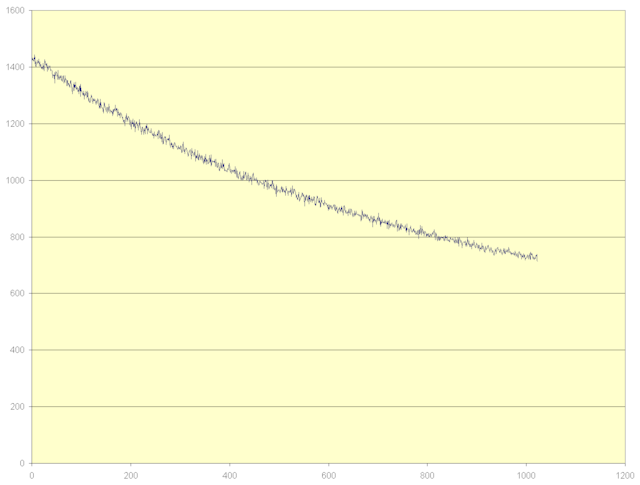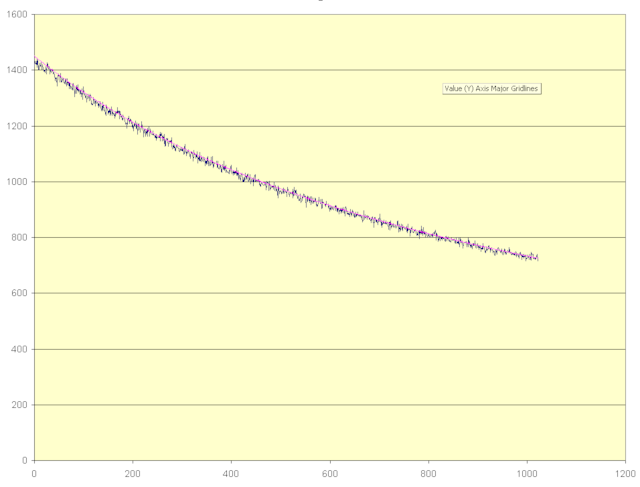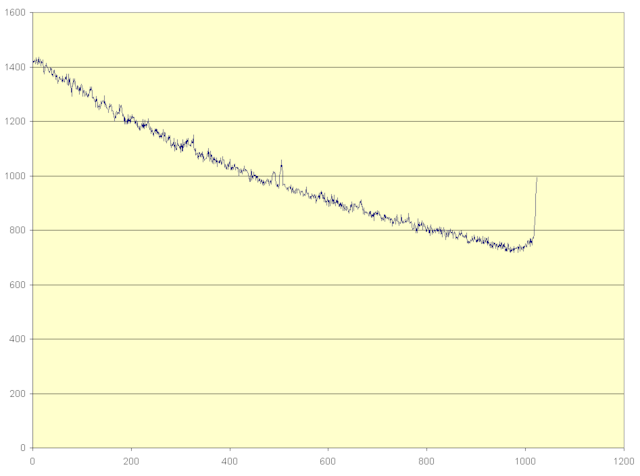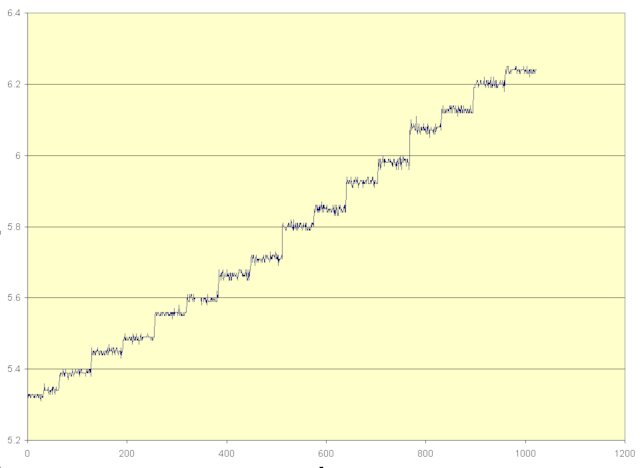# cbloom rants

## 2/10/2014

### 02-10-14 - Understanding ANS - 9

If you just want to understand the basics of how ANS works, you may skip this post. I'm going to explore some unsolved issues about the sort order.

Some issues about constructing the ANS sort order are still mysterious to me. I'm going to try to attack a few points.

One thing I said wrote last time needs some clarification - "Every slot has an equal probability of 1/M."

What is true is that every character of the output string is equiprobable (assuming again that the Fs are the true probabilities). That is, if you have the string S[] with L symbols, each symbol s occurs Fs times, then you can generate symbols with the correct probability by just drawing S[i] with random i.

The output string S[] also corresponds to the destination state of the encoder in the renormalization range I = [L,2L-1]. What is not true is that all states in I are equally probable.

To explore this I did 10,000 random runs of encoding 10,000 symbols each time. I used L=1024 each time, and gathered stats from all the runs.

This is the actual frequency of the state x having each value in [1024,2047] (scaled so that the average is 1000) :The lowest most probable states (x=1024) have roughly 2X the frequency of the high least probable states (x=2047).

Note : this data was generated using Duda's "precise initialization" (my "sort by sorting" with 0.5 bias). Different table constructions will create different utilization graphs. In particular the various heuristics will have some weird bumps. And we'll see what different bias does later on.

This is the same data with 1/X through it :This probability distribution (1/X) can be reproduced just from doing this :

```
x = x*b + irandmod(b); // for any base b

while( x >= 2*K ) x >>= 1;

stats_count[x-K] ++;

```
though I'd still love to see an analytic proof and understand that better.

So, the first thing I should correct is : final states (the x' in I) are not equally likely.

How that should be considered in sort construction, I do not know.

The other thing I've been thinking about was why did I find that the + 1.0 bias is better in practice than the + 0.5 bias that Duda suggests ("precise initialization") ?

What the +1 bias does is push low probability symbols further towards the end of the sort order. I've been contemplating why that might be good. The answer is not that the end of the sort order makes longer codelens, because that kind of issue has already been accounted for.

My suspicion was that the +1 bias was beating the +0.5 bias because of the difference between normalized counts and unnormalized original counts.

Recall that to construct the table we had to make normalized frequences Fs that sum to L. These, however, are not the true symbol frequencies (except in synthetic tests). The true symbol frequencies had to be scaled to sum to L to make the Fs.

The largest coding error from frequency scaling is on the least probable symbols. In fact the very worst case is symbols that occur only once in a very large file. eg. in a 1 MB file a symbol occurs once; its true probability is 2^-20 and it should be coded in 20 bits. But we scale the frequencies to sum to 1024 (for example), it still must get a count of 1, so it's coded in 10 bits.

What the +1 bias does is take the least probable symbols and push them to the end of the table, which maximizes the number of bits they take to code. If the {Fs} were the true frequencies, this would be bad, and the + 0.5 bias would be better. But the {Fs} are not the true frequencies.

This raises the question - could we make the sort order from the true frequencies instead of the scaled ones? Yes, but you would then have to either transmit the true frequencies to the decoder, or transmit the sort order. Either way takes many more bits than transmitting the scaled frequencies. (in fact in the real world you may wish to transmit even approximations of the scaled frequencies). You must ensure the encoder and decoder use the same frequencies so they build the same sort order.

Anyway, I tested this hypothesis by making buffers synthetically by drawing symbols from the {Fs} random distribution. I took my large testset, for each file I counted the real histogram, made the scaled frequencies {Fs}, then regenerated the buffer from the frequencies {Fs} so that the statistics match the data exactly. I then ran tANS on the synthetic buffers and on the original file data :

```
synthetic data :

total bytes out : 146068969.00  bias=0.5
total bytes out : 146117818.63  bias=1.0

real data :

total bytes out : 144672103.38  bias=0.5
total bytes out : 144524757.63  bias=1.0

```
On the synthetic data, bias=0.5 is in fact slightly better. On the real data, bias=1.0 is slightly better. This confirms that the difference between the normalized counts & unnormalized counts is in fact the origin of 1.0's win in my previous tests, but doesn't necessarily confirm my guess for why.

An idea for an alternative to the bias=1 heuristic is you could use bias=0.5 , but instead of using the Fs for the sort order, use the estimated original count before normalization. That is, for each Fs you can have a probability model of what the original count was, and select the maximum-likelihood count from that. This is exactly analoguous to restoring to expectation rather than restoring to middle in a quantizer.

Using bias=1.0 and measuring state occurance counts, we get this :Which mostly has the same 1/x curve, but with a funny tail at the end. Note that these graphs are generated on synthetic data.

I'm now convinced that the 0.5 bias is "right". It minimizes measured output len on synthetic data where the Fs are the true frequencies. It centers each symbol's occurances in the output string. It reproduces the 1/x distribution of state frequencies. However there is still the missing piece of how to derive it from first principles.

BTW

While I was at it, I gathered the average number of bits output when coding from each state. If you're following along with Yann's blog he's been explaining FSE in terms of this. tANS outputs bits to get the state x down into the coding range Is for the next symbol. The Is are always lower than I (L), so you have to output some bits to scale down x to reach the Is. x starts in [L,2L) and we have to output bits to reach [Fs,2Fs) ; the average number of bits required is like log2(L/Fs) which is log2(1/P) which is the code length we want. Because our range is [L,2L) we know the average output bit count from each state must differ by 1 from the top of the range to the bottom. In fact it looks like this :Another way to think about it is that at state=L , the state is empty. As state increases, it is holding some fractional bits of information in the state variable. That number of fraction bits goes from 0 at L up to 1 at 2L.

Ryg just pointed me at a proof of the 1/x distribution in Moffat's "Arithmetic Coding Revisited" (DCC98).

The "x" in ANS has the same properties as the range ("R") in an arithmetic coder.

The bits of information in x is I ~= log( x )

I is in [0,1] and is a uniform random value, Pr(I) ~= 1

if log(x) has Pr ~= 1 , then Pr(x) must be ~= 1/x

The fact that I is uniform is maybe not entirely obvious; Moffat just hand-waves about it. Basically you're accumulating a random variable into I ( -log2(P_sym) ) and then dropping the integer part; the result is a fractional part that's random and uniform.

Jarek Duda said...

Hi Charles,
I think your "bias 1 works better for the real data" corresponds to the fact that in real data there happen extremely low probable symbols - bias 1/2 approximates them as 1/L, while bias 1 a bit lower.
If we encode p_s sequence with encoder optimal for q_s distribution, the least probable symbols are the most significant:
deltaH ~ sum_s (p_s-q_s)^2/p_s

So for a good initializer, I would first take the extremely low probable symbols (let say < 0.5/L) as a singletons to the end of the table, and then use precise initialization for the remaining ones.
Additionally, as f[s]/L ~ p[s] quantization is not exact, for example the "bias" could be individually chosen: as a bit larger if p[s] < f[s]/L, or smaller otherwise.
... but the details need further work.
Best,
Jarek

Cyan said...

I find the 1/x distribution picture very interesting. Maybe there could be a way to use that in calculating more precisely distribution weight.

Cyan said...

I tried to reproduce your result, but so far got only a hint that it was the "general direction", not something as clean as your distribution picture.

Some differences :
- I'm using real-world input, not synthetic
- FSE distribution is not the same as yours, and is likely introducing some noise & distortion.

Anyway, it shows that the probability of a state is not necessarily a clean 1/X distribution graph, and will vary a lot depending on symbol distribution over the state table, something you hinted into your second graph.

cbloom said...

Yes, you only get the perfect 1/x when the statistics of the data match the normalized counts exactly (eg. with synthetic data), and when the sort is "Duda's precise".

I still don't entirely understand the 1/x.

I'd like to see a proof that the optimal sort order must lead to a 1/x distribution, or vice-versa that a 1/x distribution must provide minimum code length.

Pascal said...

note that you need to make sure that 'b' is not a power of 2. Otherwise, 'x' will be constant.

/skal

Pascal said...

and, for the record, here is a C-version of the iteration, showing the emerging 1/x stationary distribution:

http://pastebin.com/NPqmsR8L